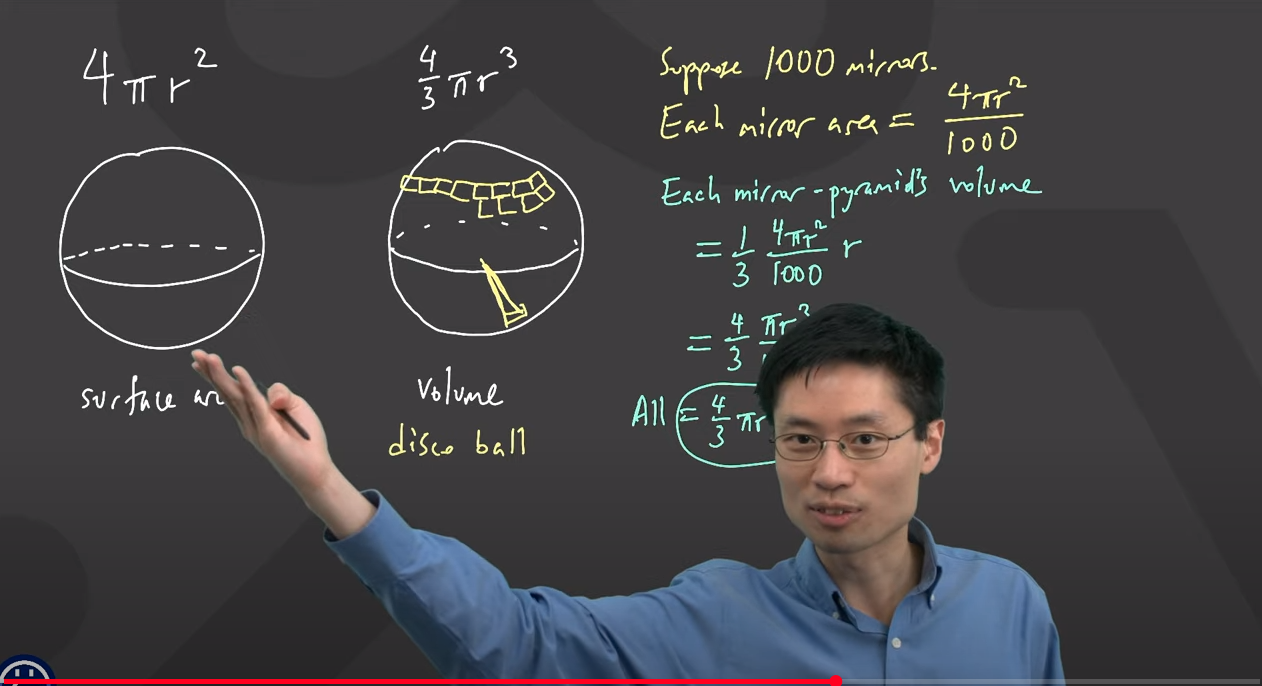# Volume of a sphere

• Why is the volume of a sphere
$$\frac{4}{3}πr^3$$ ?

• Hi @RZ923!

Nice question! Not so many people even wonder about where this formula came from! However, to be able to prove the formula of the volume of a sphere, you should know about integrals, how to use them and where they came from. Before you reach that point, you will first gain lots of other knowledge and experience. Then you will be even able to prove it by yourself.

• @nastya thanks• @rz923 I myself am not a mathematics whiz but I'm pretty sure it involves fitting the largest sphere, given a cylinder.

• @energizedpanda In the March 26 Ask Math Anything livestream, Prof. Loh actually explained why the volume of a sphere is $$\frac{1}{3}^{\text{rd}}$$ its surface area!

It has to do with the fact that you can chop the volume into infinitesimally-small pyramids where the bases lie on the surface of the sphere and the opposite corners all lie at the center of the sphere. Then, since the volume of a pyramid is just $$\frac{1}{3} \text{ ( area of base ) } \times \text{ height },$$ you just take the surface area, multiply by the height of each pyramid, which is the same for each pyramid, $$r,$$ and multiply by $$\frac{1}{3}$$ to get the volume of the sphere!